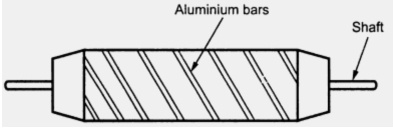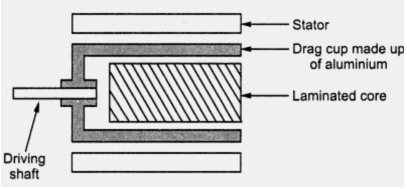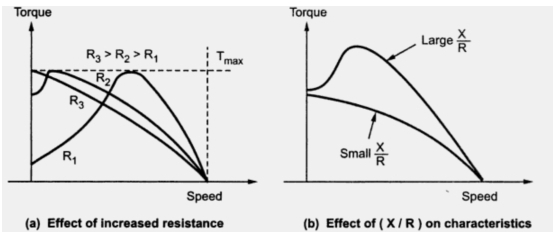### A.C. Servomotor

Servomotor Part 4
Most of the servomotors used in the low power servomechanism are a.c. servomotors. The a.c. servomotor is basically two phase induction motor. The output power of a.c. servomotor varies from fraction of watts to few hundred of watts. The operating frequency is 50 Hz to 400 Hz.

1.1 Construction
The a.c. servomotor is basically consists of a stator and a rotor. The stator carries two windings, uniformly distributed and displaced by 90o in space, from each other.
On winding is called as main winding or fixed winding or reference winding. The reference winding is excited by a constant voltage a.c. supply.
The other winding is called as control winding. It is excited by variable control voltage, which is obtained from a servoamplifier. The winding are 90o  away from each other and control voltage is 90o  out of phase with respect to the voltage applied to the reference winding. This is necessary to obtain rotating magnetic field.
The schematic stator is shown in the Fig. 1.Fig. 1 Stator of a.c. servomotor

To reduce the loading on the amplifier, the input impedance i.e. the impedance of the control winding in increased by using a tuning capacitor in parallel with the control winding.
1.2 Rotor
The rotor is generally of two types. The two types of rotors are,
1. Squirrel cage rotor
2. Drag cup type rotor
1.2.1 Squirrel Cage Rotor
The usual squirrel cage rotor has aluminimum  bars which are shorted at the ends with the help of the end rings. The overall construction looks like a cage. The construction is similar to the squirrel cage rotor used for the three phase induction motors.
This has small diameter and large length. This is because to reduce the inertia. Aluminimum conductors are used to keep weight small. Its resistance is high to keep torque-speed characteristics as linear as possible. Air gap is kept very small which reduces the magnetism current. The cage type of rotor uses the skewed bars as shown in the Fig. 2.Fig. 2  Cage type rotor construction

To reduce the inertia further, a drag cup type of rotor construction is used. There are two air gaps in this construction. The drag cup is made up of nonmagnetic material like copper, aluminium or an alloy. The slotted rotor laminations in this construction. These are wound for as many number of poles as possible so that operating speed of motor is very low. Such a construction is used in very low power applications. A drag cup type rotor construction is shown in the Fig. 3.Fig. 3  Drag cup type rotor construction

1.3 Operating Principle
The operating principle of two phase a.c. servomotor is same as that of normal three phase induction motor. The control voltage applied to the control winding and the voltage applied to the reference winding are 90o  out of phase. Hence the flux produces by current through control winding is also 90o out of phase with respect to the flux produced by the current through the reference winding. The resultant flux in the air gap is hence rotating flux sweeps over the rotor, the e.m.f. gets induced in the rotor. This e.m.f. circulates the current through the rotor. The rotor current produces its own flux called as rotor flux. This flux interacts with the rotating magnetic field, producing a torque on the rotor and rotor starts rotating.
In the two phase a.c. servomotors, the polarity of the control voltage determines the direction of rotation. A change in the sign of the control voltage reverses the direction of rotation of the motor. Since the reference voltage is constant, the torque and the angular speed are the functions of the control voltage.

1.4 Torque-Speed Characteristics
The usual torque-speed characteristics of an induction motor with high inductance to resistance ratio are not suitable for the servomotor. A servomotor must have
1. Linear torque-speed characteristics
2. Slope of the torque-speed characteristics must be negative.
3. The characteristics must be parallel to one another for various values of the control voltage applies.
It is seen that when rotor resistance is increased the torque-speed characteristics becomes more and more linear. In general for low inductance to resistance ratio, the torque-speed characteristics are almost linear. This is shown in the Fig. 4(a) and (b).Fig. 4

In practice, design of the motor is so as to get almost linear torque-speed characteristics. A servomotor rotates only when a detectable error occurs at low voltage and it rarely operates at high speeds. Hence for a given value of control voltage, torque-speed characteristics are almost linear. Fig. 5 shows the torque-speed characteristics for various control voltages.Fig. 5 Torque-speed characteristics of a.c  servomotor

All the characteristics are equally spaced for equal increments of control voltage as shown.

1.5 Other Performance Characteristics
The performance characteristics other than the torque-speed characteristics of a two phase a.c. servomotor are shown in the Fig. 6.Fig. 6  Performance characteristics of a typical a.c. servomotor

As torque developed increases, current drawn and the power input increases. Power factors also improves upto certain limit. While the efficiency increases upto certain limit and then starts decreasing.

1.6 Features of A.C. Servomotor
The various features of a.c. servomotor are,
1. Light in weight for quick response.
2. Robust in construction.
3. It is reliable and its operation is stable in nature.
4. Smooth and noise free operation.
5. Large torque to weight ratio.
6. Large resistance to reactance ratio.
7. No brushes or slip rings are required. Hence maintenance free.
8. Driving circuits are simple to design.
9. The negative slope of the torque-slip characteristics adds more friction improving the damping. This improves the stability of the motor. This features is called internal electric damping of two phase a.c. servomotor.

1.7 Applications of A.C. Servomotor
As mentioned earlier a.c. servomotor are preferred for low power applications. Consider an a.c. position control system where load position is to be maintained constant. The driving motor used is two phase a.c. servomotor. The potentiometer arrangement compares the actual position with the reference position to generate the error voltage. The error voltage is amplifier which generates control voltage . This voltage is applied to the control winding of two phase a.c. servomotor. This control The torque and inturn controls the output position of the load. As driving motor is a.c. servomotor, the system is called as a.c. position control system. The entire scheme is shown in the Fig. 7.Fig. 7 Application of a.c. servomotor : A.C. position control system

The other applications of a.c. servomotors are,
1. Instrument servos                  2. Process controllers           4. Robotics
4. Self balancing recorders          5. Machine tools
And is preferred for number of other applications in which precise angular motion is necessary.